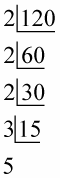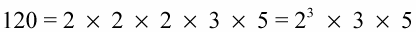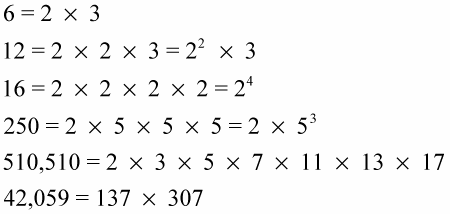##### Linear Algebra For DummiesPrime factorization shows you the only way a number can be factored. The process of prime factorization breaks down a composite number into the prime numbers that, when multiplied together, give you that composite number. Every number’s prime factorization is unique.

The opposite of prime numbers, composite numbers, can be broken down into factorable, reducible pieces. Whole numbers larger than 1 that aren’t prime are composite numbers.

A good way of writing out prime factorizations is to do an upside-down division:

1. Put a prime factor on the outside left, and the result or quotient (the number of times it divides evenly) underneath.

2. Divide the quotient (the number underneath) by another prime number, and keep doing this until the bottom number is a prime.

Then you can stop. The order you do this in doesn’t matter. You get the same result or list of prime factors no matter what order you use.

Example: Determine the prime factorization of 120.3. Use the numbers from the left to write out the prime factorization.

Look at the numbers going down the left side of the work and the number at the bottom. They act the same as the divisors in a division problem, but in this case, they’re all prime numbers. Although many composite numbers could have played the role of divisor for the number 120, the numbers for the prime factorization of 120 must be prime-number divisors.

When using this process, you usually do all the 2s first, then all the 3s, then all the 5s, and so on to make the prime factorization process easier, but you can do this in any order:Here are some examples of how to write out the prime factorizations of composite numbers:The factors of some numbers aren’t always obvious. Finding the prime factorization of numbers like the last example above without a calculator, computer, or list of primes is difficult.